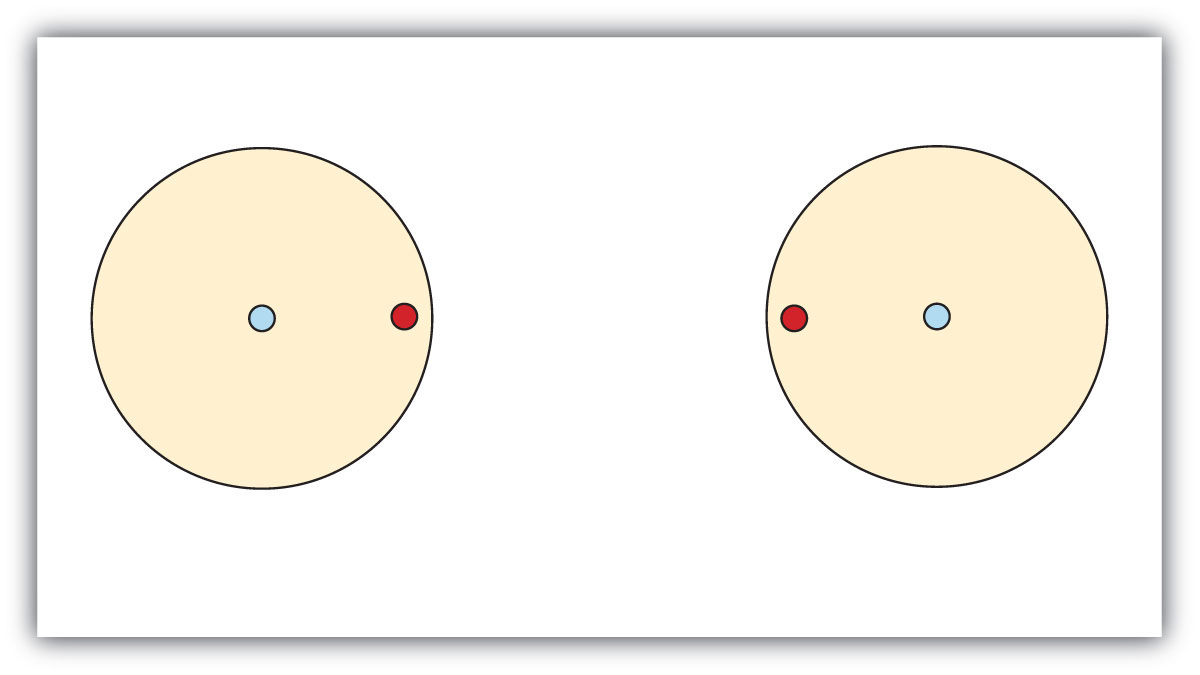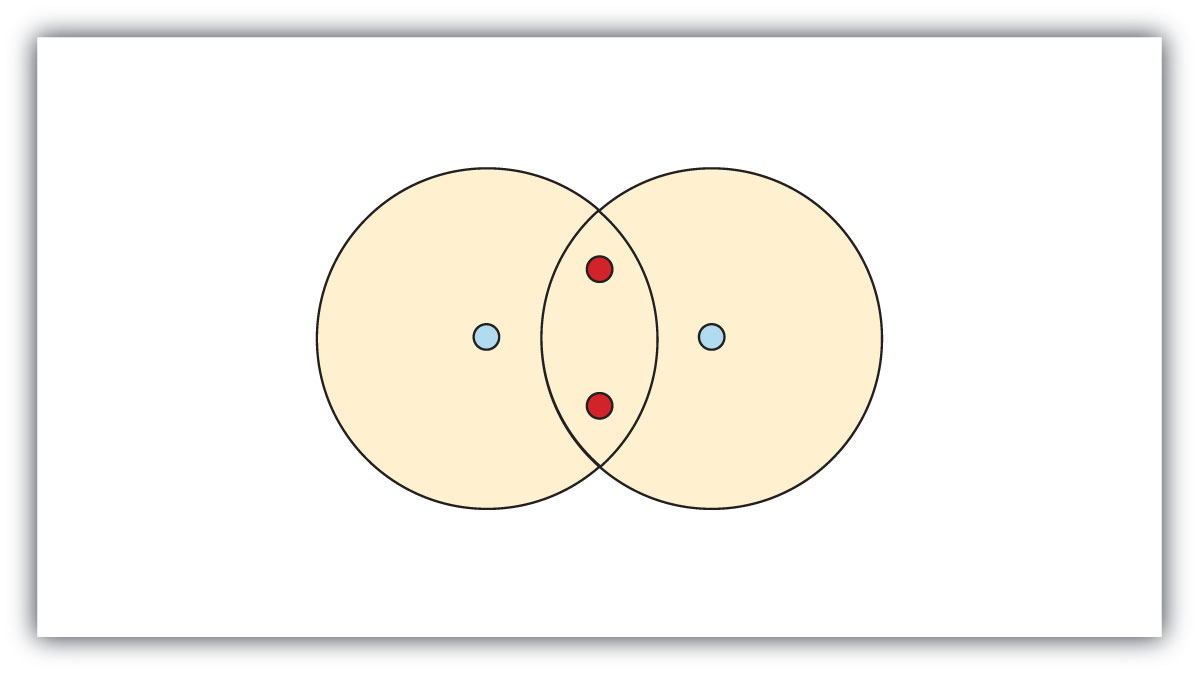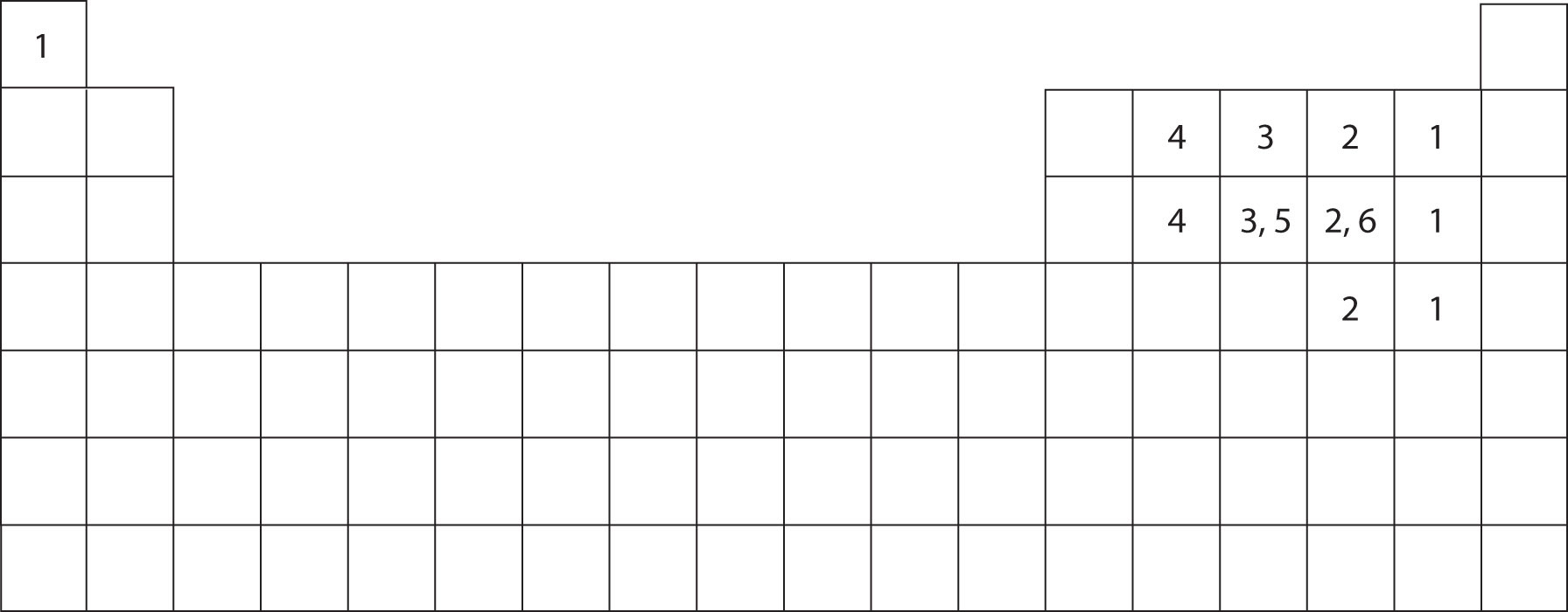This is “Covalent Bonds”, section 4.1 from the book Introduction to Chemistry: General, Organic, and Biological (v. 1.0). For details on it (including licensing), click here.

Has this book helped you? Consider passing it on:
Creative Commons supports free culture from music to education. Their licenses helped make this book available to you.
DonorsChoose.org helps people like you help teachers fund their classroom projects, from art supplies to books to calculators.

## 4.1 Covalent Bonds

### Learning Objective

1. Describe how a covalent bond forms.

You have already seen examples of substances that contain covalent bonds. One substance mentioned in Chapter 3 "Ionic Bonding and Simple Ionic Compounds" was water (H2O). You can tell from its formula that it is not an ionic compound; it is not composed of a metal and a nonmetal. Consequently, its properties are different from those of ionic compounds.

## Electron Sharing

Chapter 3 "Ionic Bonding and Simple Ionic Compounds" described how electrons can be transferred from one atom to another so that both atoms have an energy-stable outer electron shell. Because most filled electron shells have eight electrons in them, chemists called this tendency the octet rule. But there is another way an atom can achieve a full valence shell: atoms can share electrons.

This concept can be illustrated by using two hydrogen atoms, each of which has a single electron in its valence shell. (For small atoms such as hydrogen atoms, the valence shell will be the first shell, which holds only two electrons.) We can represent the two individual hydrogen atoms as follows:In contrast, when two hydrogen atoms get close enough together to share their electrons, they can be represented as follows:By sharing their valence electrons, both hydrogen atoms now have two electrons in their respective valence shells. Because each valence shell is now filled, this arrangement is more stable than when the two atoms are separate. The sharing of electrons between atoms is called a covalent bondThe sharing of electrons between two atoms., and the two electrons that join atoms in a covalent bond are called a bonding pair of electronsThe two electrons that join atoms in a covalent bond.. A discrete group of atoms connected by covalent bonds is called a moleculeA discrete group of atoms connected by covalent bonds.—the smallest part of a compound that retains the chemical identity of that compound.

Chemists frequently use Lewis diagrams to represent covalent bonding in molecular substances. For example, the Lewis diagrams of two separate hydrogen atoms are as follows:The Lewis diagram of two hydrogen atoms sharing electrons looks like this:This depiction of molecules is simplified further by using a dash to represent a covalent bond. The hydrogen molecule is then represented as follows:Remember that the dash, also referred to as a single bondA covalent bond formed by a single pair of electrons., represents a pair of electrons.

The bond in a hydrogen molecule, measured as the distance between the two nuclei, is about 7.4 × 10−11 m, or 74 picometers (pm; 1 pm = 1 × 10−12 m). This particular bond lengthThe distance between two nuclei in a covalent bond. represents a balance between several forces: the attractions between oppositely charged electrons and nuclei, the repulsion between two negatively charged electrons, and the repulsion between two positively charged nuclei. If the nuclei were closer together, they would repel each other more strongly; if the nuclei were farther apart, there would be less attraction between the positive and negative particles.

Fluorine is another element whose atoms bond together in pairs to form diatomic (two-atom) molecules. Two separate fluorine atoms have the following electron dot diagrams:Each fluorine atom contributes one valence electron, making a single bond and giving each atom a complete valence shell, which fulfills the octet rule:The circles show that each fluorine atom has eight electrons around it. As with hydrogen, we can represent the fluorine molecule with a dash in place of the bonding electrons:Each fluorine atom has six electrons, or three pairs of electrons, that are not participating in the covalent bond. Rather than being shared, they are considered to belong to a single atom. These are called nonbonding pairs (or lone pairs)Electron pair that does not participate in covalent bonds. of electrons.

## Covalent Bonds between Different Atoms

Now that we have looked at electron sharing between atoms of the same element, let us look at covalent bond formation between atoms of different elements. Consider a molecule composed of one hydrogen atom and one fluorine atom:Each atom needs one additional electron to complete its valence shell. By each contributing one electron, they make the following molecule:In this molecule, the hydrogen atom does not have nonbonding electrons, while the fluorine atom has six nonbonding electrons (three lone electron pairs). The circles show how the valence electron shells are filled for both atoms.

### Example 1

Draw the Lewis diagram for each compound.

1. a molecule composed of two chlorine atoms
2. a molecule composed of a hydrogen atom and a bromine atom

Solution

1. Chlorine has the same valence shell electron configuration as fluorine, so the Lewis diagram for a molecule composed of two chlorine atoms is similar to the one for fluorine:2. Bromine has the same valence shell electron configuration as fluorine, so the Lewis diagram for a molecule composed of a hydrogen atom and a bromine atom is similar to that for hydrogen and fluorine:### Skill-Building Exercise

Draw the Lewis diagram for each compound.

1. a molecule composed of one chlorine atom and one fluorine atom

2. a molecule composed of one hydrogen atom and one iodine atom

Larger molecules are constructed in a similar fashion, with some atoms participating in more than one covalent bond. For example, water, with two hydrogen atoms and one oxygen atom, and methane (CH4), with one carbon atom and four hydrogen atoms, can be represented as follows:Atoms typically form a characteristic number of covalent bonds in compounds. Figure 4.2 "How Many Covalent Bonds Are Formed?" shows the number of covalent bonds various atoms typically form.

Figure 4.2 How Many Covalent Bonds Are Formed?In molecules, there is a pattern to the number of covalent bonds that different atoms can form. Each block with a number indicates the number of covalent bonds formed by that atom in neutral compounds.

### Concept Review Exercises

1. How is a covalent bond formed between two atoms?

2. How does covalent bonding allow atoms in group 6A to satisfy the octet rule?

1. Covalent bonds are formed by two atoms sharing electrons.

2. The atoms in group 6A make two covalent bonds.

### Key Takeaway

• A covalent bond is formed between two atoms by sharing electrons.

### Exercises

1. Define covalent bond.

2. What is electron sharing?

3. Draw the Lewis diagram for the covalent bond in the Br2 molecule.

4. Draw the Lewis diagram for the covalent bond in the I2 molecule.

5. Draw the Lewis diagram for the covalent bond in the HCl molecule.

6. Draw the Lewis diagram for the covalent bond in the HI molecule.

7. What is the difference between a molecule and a formula unit?

8. Why do hydrogen atoms not follow the octet rule when they form covalent bonds?

9. Draw the Lewis diagram for the covalent bonding in H2S. How many bonding electrons and nonbonding electrons are in the molecule?

10. Draw the Lewis diagram for the covalent bonding in NI3. How many bonding electrons and nonbonding electrons are in the molecule?

11. Draw the Lewis diagram for the covalent bonding in CF4. How many bonding electrons and nonbonding electrons are in the molecule?

12. Draw the Lewis diagram for the covalent bonding in PCl3. How many bonding electrons and nonbonding electrons are in the molecule?

13. How many covalent bonds does a hydrogen atom typically form? Why?

14. How many covalent bonds does an oxygen atom typically form? Why?

15. Tellurium atoms make covalent bonds. How many covalent bonds would a tellurium atom make? Predict the formula of a compound between tellurium and hydrogen.

16. Tin atoms make covalent bonds. How many covalent bonds would a tin atom make? Predict the formula of a compound between tin and hydrogen.

17. Astatine is a synthetic element, made one atom at a time in huge “atom-smasher” machines. It is in the halogen group on the periodic table. How many covalent bonds would an atom of this element form?

18. There have been reports that atoms of element 116 were made by smashing smaller atoms together. Using the periodic table, determine what column element 116 would be in and suggest how many covalent bonds an atom of this element would form.

1. A covalent bond is formed when two atoms share electrons.

2.3.4. A molecule is a discrete combination of atoms; a formula unit is the lowest ratio of ions in a crystal.

5.bonding electrons: 4; nonbonding electrons: 4

6.bonding electrons: 8; nonbonding electrons: 24

7. Hydrogen atoms form only one covalent bond because they have only one valence electron to pair.

8. two; H2Te

9. one